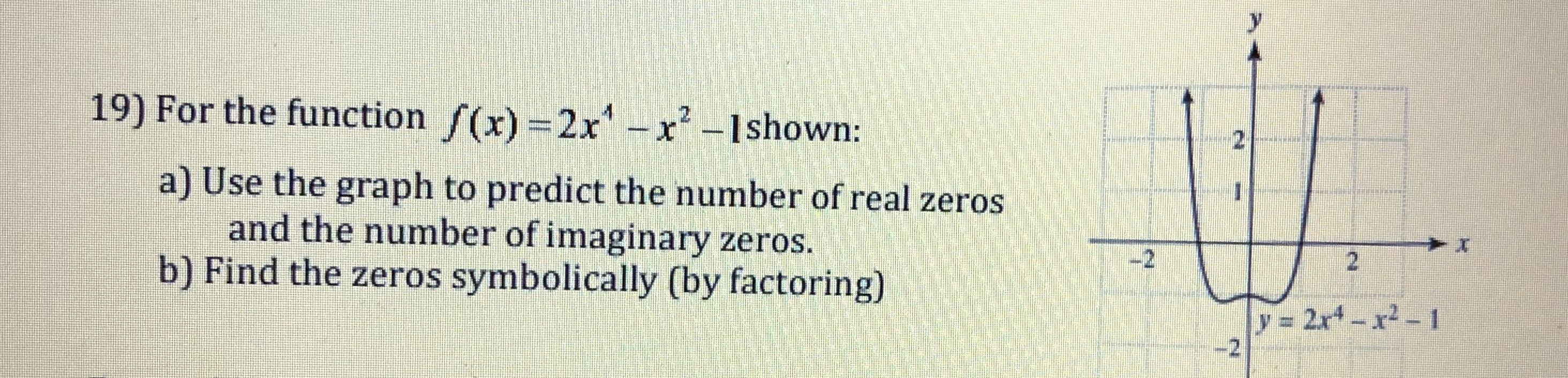19) For the function f(x)-2x-shown:a) Use the graph to predict the number of real zerosand the number of imaginary zeros.b) Find the zeros symbolically (by factoring)-2

Questionhelp_outlineImage Transcriptionclose19) For the function f(x)-2x-shown: a) Use the graph to predict the number of real zeros and the number of imaginary zeros. b) Find the zeros symbolically (by factoring) -2 fullscreen
Step 1

(1)

The given function is f(x) = 2x4 x2 – 1.

Step 2

(2)

Factorize the function f(x) = 2x4 x2 – 1 as follows.

Step 3

Obtain the zeros of the function f(x) = 2x4 – x2...

Want to see the full answer?

See Solution

Want to see this answer and more?

Our solutions are written by experts, many with advanced degrees, and available 24/7

See Solution
Tagged in

Other